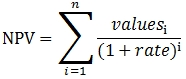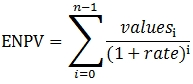SQL Server NPV function

NPV

Updated: 07 February 2011

Note: This documentation is for the SQL2008 (and later) version of this XLeratorDB function, it is not compatible with SQL Server 2005.

Use NPV to calculate the net present value of an investment based on a series of periodic cash flows and a discount rate. NPV is closely related to ENPV function.

If n is the number of cash flows in the list of values, the formula for NPV is:Whereas the formula for ENPV is:Where
N = Number of cashflows
Values = Cashflow amounts
Rate = Discount rate

Syntax
SELECT [westclintech].[wct].[NPV] (
<@Rate, float,>
,<@CF_Amt, float,>
,<@Per, int,>)
Arguments
@Rate
the rate to be used for discounting the cash flows in calculating the net present value. @Rate is an expression of type float or of a type that can be implicitly converted to float.
@CF_Amt
the cash flow amounts. @CF_Amt is an expression of type float or of a type that can be implicitly converted to float.
@Per
the period in which the cash flow occurred. @Per is an expression of type int or of a type that can be implicitly converted to int.
Return Type
float
Remarks
·         The NPV aggregate function requires a series of cash flows (@CF_Amt) and the periods in which those cash flows occurred (@Per) as input. As a result, the order of the cash flows is not important.
·         Periods in which the cash flow is zero, or in which there is no cash flow, do not have to be included.
·         The periods can start and end with any integer value, including negative numbers.
·         There can be multiple cash flows with the same period number.
·         The discount rate (@Rate) must remain constant for the aggregate GROUP.
·         If the discount rate (@Rate) is equal to -1, a NULL will be returned.
·         It is important to be consistent with the units for @Rate and @Per. For example if payments are to be paid monthly, then @Rate should be the monthly rate, which can be specified as the annual rate divided by 12. If payments are made quarterly, divide the annual rate by 4. If payments are made semi-annually, divide the annual rate by 2.
·         Funds that are paid should be represented with negative numbers. Funds that are received should be represented as positive numbers.
·         For calculations involving dates, consider using the XNPV aggregate function.
·         The NPV function differs from the ENPV function in that the ENPV function calculates the discount rate as (1+rate)i for i equal zero to n-1, where n is the difference between the maximum period value and the minimum period value.   The NPV function calculates the discount rate as (1+rate)i for i equal one to n, where n is the difference between the maximum period value and the minimum period value. The ENPV result divided by the NPV result should be equal to 1 plus the rate (@Rate).
Examples
In this example we do not have cash flows occuring in every period and we have two cash flows occuring in period 14
SELECT wct.NPV(.0075, cf_amt, per) as NPV
FROM (VALUES
(-10000, 1),
(2000, 2),
(1500, 5),
(3000, 6),
(3800, 10),
(2500, 14),
(2500, 14)
) n(cf_amt, per)
This produces the following result.
NPV
----------------------
4388.04234280299

(1 row(s) affected)

In this example, we will use the same cash flows as the previous example, with the same intervals between the cash flows, but instead of starting at period 1, we will start at period 101.
SELECT wct.NPV(.0075, cf_amt, per) as NPV
FROM (VALUES
(-10000, 101),
(2000, 102),
(1500, 105),
(3000, 106),
(3800, 110),
(2500, 114),
(2500, 114)
) n(cf_amt, per)
This produces the following result.
NPV
----------------------
4388.04234280299

(1 row(s) affected)

In this example, we will use the same cash flows, with the same spacing among periods, but we will use negative period numbers and the cash flows will not be in order in the derived table.
SELECT wct.NPV(.0075, cf_amt, per) as NPV
FROM (VALUES
(2500, -86),
(2500, -86),
(3800, -90),
(3000, -94),
(1500, -95),
(2000, -98),
(-10000, -99)
) n(cf_amt, per)
This produces the following result.
NPV
----------------------
4388.04234280299

(1 row(s) affected)

In this example, the period numbers are not stored in a table, but have to be calculated as an input into the function. We will store the cash flows by year and month.
SELECT wct.NPV(.0075, cf, yr*12 + mth) as NPV
FROM (VALUES
(2011,1,-10000),
(2011,2,2000),
(2011,5,1500),
(2011,6,3000),
(2011,10,3800),
(2012,2,2500),
(2012,2,2500)
) n(yr, mth, cf)
This produces the following result.
NPV
----------------------
4388.04234280299

(1 row(s) affected)

In this example, we will add another column to the derived table from the previous example, which will allow for a different rate for each period. This will return an error message, as the function requires that the rate value be the same for all cash flows.
SELECT wct.NPV(rate, cf, yr*12 + mth) as NPV
FROM (VALUES
(2011,1,-10000, .0075),
(2011,2,2000, .0075),
(2011,5,1500, .0075),
(2011,6,3000, .0075),
(2011,10,3800, .0075),
(2012,2,2500, .0080),
(2012,2,2500, .0080)
) n(yr, mth, cf, rate)
This produces the following message and will not return a result.
Msg 6522, Level 16, State 2, Line 1
A .NET Framework error occurred during execution of user-defined routine or aggregate "ENPV":
XLeratorDB_financial2008.Core.Exceptions+Function_AggregateException:
[Product version 1.7 Build: 0126.208]
An error occurred in Aggregate Function. (Accumulate) - Data is Null. This method or property cannot be called on Null values.
Function: ENPV
Passed Parameters:
'Rate' = System.Data.SqlTypes.SqlDouble:Null
'CF_Amt' = System.Data.SqlTypes.SqlDouble:7255
'Per' = System.Data.SqlTypes.SqlInt32:5

Internal refs:
'd_rate' = System.Double:-1
'disc_amt' = System.Double:0
'start_per' = System.Int32:2147483647
*** Extra Information: Data is Null. This method or property cannot be called on Null values.
XLeratorDB_financial2008.Core.Exceptions+Function_AggregateException:
at XLeratorDB_financial2008.ENPV.HandleException(String Method, String Ref, ENPV& Merge, FunctionParamsClass& Params, Exception& Ex)
at XLeratorDB_financial2008.ENPV.Accumulate(SqlDouble Rate, SqlDouble CF_Amt, SqlInt32 Per)
.

If you want to calculate the present value of future cash flows using different rates for different periods, you can use the SUM function with the NPV function.
In this example, we set the rate equal to -1.
SELECT wct.NPV(-1.0, cf_amt, per) as NPV
FROM (VALUES
(-10000, 1),
(2000, 2),
(1500, 5),
(3000, 6),
(3800, 10),
(2500, 14),
(2500, 14)
) n(cf_amt, per)
This produces the following result.
NPV
----------------------
NULL

(1 row(s) affected)

NPV is an AGGREGATE function and supports all the syntax available to any SQL Server AGGREGATE function. Here is an example using a GROUP BY.
SELECT region
,wct.NPV(.015
,cf
,(yr-2011)*4+quar) as NPV
FROM (
VALUES
('New England',2011,1,9589),
('New England',2011,2,5866),
('New England',2011,3,9530),
('New England',2011,4,6805),
('New England',2012,1,2567),
('New England',2012,2,9403),
('New England',2012,3,5607),
('New England',2012,4,2469),
('MidAtlantic',2011,1,4366),
('MidAtlantic',2011,2,5488),
('MidAtlantic',2011,3,5955),
('MidAtlantic',2011,4,5450),
('MidAtlantic',2012,1,7255),
('MidAtlantic',2012,2,2458),
('MidAtlantic',2012,3,1738),
('MidAtlantic',2012,4,3866),
('Southeast',2011,1,1671),
('Southeast',2011,2,4383),
('Southeast',2011,3,4913),
('Southeast',2011,4,8209),
('Southeast',2012,1,1476),
('Southeast',2012,2,3056),
('Southeast',2012,3,3931),
('Southeast',2012,4,5476)
) n(region, yr, quar, cf)
GROUP BY region
This prodcues the following result.
region                         NPV
----------- ----------------------
MidAtlantic       34438.5903986833
New England       48892.5575668572
Southeast         30901.4872083572

(3 row(s) affected)

SupportCopyright 2008-2019 Westclintech LLC         Privacy Policy        Terms of Service